# The difference between static pressure, dynamic pressure and total pressure?

## The difference between static pressure, dynamic pressure and total pressure?

Industrial Fans

It is the impossible to dimension a fan as long the next four parameters are not known:

• The volume flow needed for the application
• The total pressure generated by the fan
• The temperature at the air inlet
• The way the fan is connected to the system

The volume flow, the temperature and the connection can be easily determined. The total pressure gain however needs some more clarification, especially its relation to the connection method.

### Total pressure gain

The total pressure gain is the difference between the total pressure at the inlet (1) and the one at the outlet (2) of the fan. This pressure causes the airstream.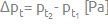Below we will see that this total pressure gain is dependent on the connection method.

#### What is total pressure?

Total pressure is the addition of all static pressures and the dynamic pressure.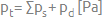#### What is static pressure?

Static pressure is the pressure applied by the air to the walls perpendicular on the airstream.  It corresponds to the sum of all pressure drops of the ducts system and its components.

#### What is dynamic pressure?

Dynamic pressure is the kinetic energy of the air that passes through the fan. It is applied to the walls that obstruct the airstream. This dynamic pressure is directly dependant on the airspeed (c) and can be calculated as: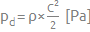As you can see, dynamic pressure is also dependant on the density and as such also on the temperature. Density is calculated as: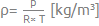p = Atmospheric pressure (standard 101325 pa)
R = gas constant for air (287 J/(kg.K)
T = Temperature in Kelvin (= 273.15 + t in °C)

From the above relations, we can deduct the following equation: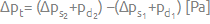As we can consider the static pressure at the inlet as vacuum pressure, it can be calculated as negative.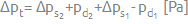### Total pressure in function of the connection method

The connection method has a direct influence on the total pressure gain.

#### Connection method A

The fan has an open inlet and open outlet.

#### Connection method B

The fan has an open inlet and a connected outlet.

#### Connection method C

The fan has a connected inlet and an open outlet.

#### Connection method D

The fan is connected at both sides.

As indicated above, the total pressure gain is calculated as: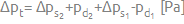For two of the four connection methods it can be simplified.

In the case of method B, the inlet is open. As a consequence, we can consider: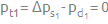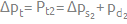In the case of method C, the outlet is open. As a consequence, we can consider: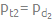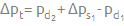If the inlet surface equals the surface of the outlet, both dynamic pressures are the same and will  nullify each: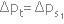### Conclusion

It is important to know the situation in which the fan is built and to know the construction of the fan (inlet and outlet dimensions), because this defines whether the static pressure is smaller, equal or higher than the total pressure.

Are you struggling to calculate pressure? Or do you have another problem with your fans? Give us a call and our team will be happy to assist you with their knowledge and expertise.